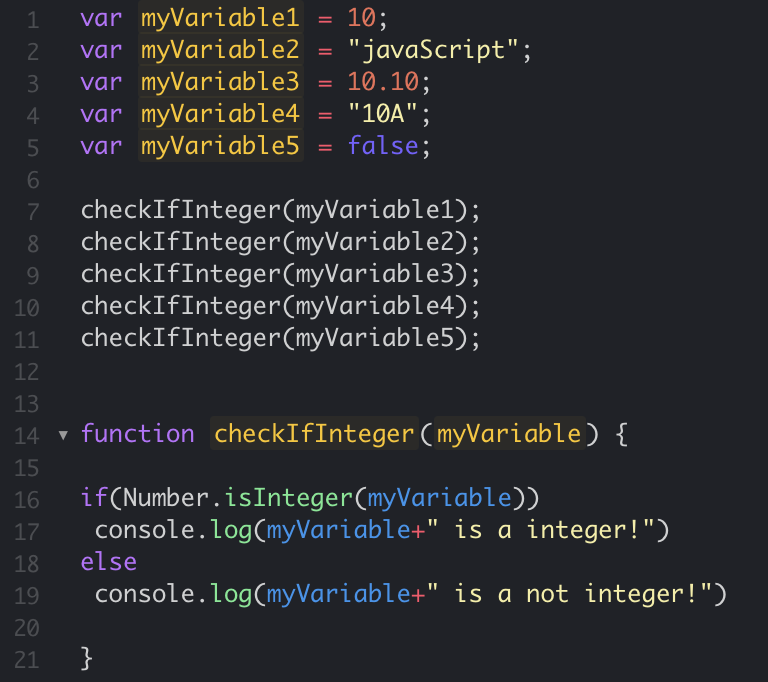# JavaScript: Check if variable is a number

In JavaScript there are multiple ways you can check if a variable is a number or not, we will see four examples,

1. Using function isNaN()
2. Using typeof operator
3. Using regular expression
4. Using Number.isInteger() function (specifically integer type check)

## Example 1: Using isNaN() function

``````var number1 = 10;
var number2 = "10a";
var number3 = "10";
var number4 = 10.1;

console.log(number1+" is a number? " + !isNaN(number1));
console.log(number2+" is a number? " + !isNaN(number2));
console.log(number3+" is a number? " + !isNaN(number3));
console.log(number4+" is a number? " + !isNaN(number4));``````
Output:
``````"10 is a number? true"
"10a is a number? false"
"10 is a number? true"
"10.1 is a number? true"``````
JSFiddle: https://jsfiddle.net/code2care/6abydkv9/7/

## Example 2: Using typeof operator

``````var number1 = 1234;
var number2 = "101bac";
var number3 = "abc";
var number4 = "10.1123";

console.log(number1+" is a " + typeof number1);
console.log(number2+" is a " + typeof number2);
console.log(number3+" is a " + typeof number3);
console.log(number4+" is a " + typeof number4);``````
Output:
``````"1234 is a number"
"101bac is a string"
"abc is a string"
"10.1123 is a string"``````

## Example 3: Using regular expression:

``````
html:
======
<br/>
<h1>
Check if inputed text is a number using Regex
</h1>
<input type="text" id="text">
<button type="button" onClick="checkisNumber()">Check</button>
<div id="result"></div>

javaScript:
========
function checkisNumber() {
var no = document.getElementById("text").value;

var isNoRegex=/^\d*(\.)?(\d{1,})?\$/;

if(!no.match(isNoRegex)) {
document.getElementById("result").innerHTML = no + " is not a number";
} else {
document.getElementById("result").innerHTML = no + " is a number";
}

}``````
JSFiddle:https://jsfiddle.net/code2care/qwtk0Lzs/9/

## Example 4: Using Number.isInteger() function:

In this example we will specifically see if the variable is of a specific number type i.e Integer

``````var myVariable1 = 10;
var myVariable2 = "JavaScript";
var myVariable3 = 10.10;
var myVariable4 = "10A";
var myVariable5 = false;

checkIfInteger(myVariable1);
checkIfInteger(myVariable2);
checkIfInteger(myVariable3);
checkIfInteger(myVariable4);
checkIfInteger(myVariable5);

function checkIfInteger(myVariable) {

if(Number.isInteger(myVariable))
console.log(myVariable+" is an integer!")
else
console.log(myVariable+" is not an integer!")

}``````
Output:

"10 is an integer!"
"JavaScript is not an integer!"
"10.1 is not an integer!"
"10A is not an integer!"
"false is not an integer!"JavaScript Check if variable is an Integer using isInteger function